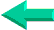### This is an excerpt from Pierre Leclerc (www.excel-vba.com)

The 2 Most Important

Excel Functions AND
Formulas

SUMPRODUCT

AND

INDEX/MATCH

## Lesson 13: SUBTOTAL Function in Excel

The Excel function SUBTOTAL is a function that can execute 11 types of calculations. SUBTOTAL is a very useful formula for analysis of data directly from  the data sheet. It will sum, count, calculate average....of the records that have been filtered in a database.

The syntax is very easy:
=SUBTOTAL(9,A2:A50))

In plain English: Execute calculation number 9 (sum) on all filtered records of range A2 to A50. If no filter is applied the calculation is executed using  all the cells in the range.

Calculation types:
1: average, 2: count (numbers), 3: count (non blanks), 4: maximum, 5: minimum, 6: product, 7: standard deviation (sample), 8: standard deviation (population), 9: sum, 10: variance (sample), 11: variance (population)

Types 7, 8, 10 and 11 are for users interested in statistics. As for type 6...

SUMPRODUCT  ¦  INDEX/MATCH  ¦  SUBTOTAL  ¦  ISERROR  ¦  HLOOKUP  ¦  LOOKUP  ¦  VLOOKUP
AVERAGEIF  ¦  AVERAGEIFS ¦  COUNTIF  ¦  COUNTIFS  ¦  SUMIF  ¦  SUMIFS  ¦  IFERRORBack home